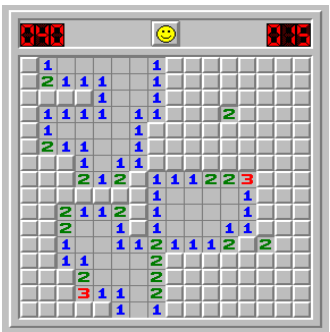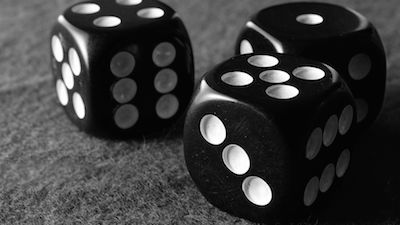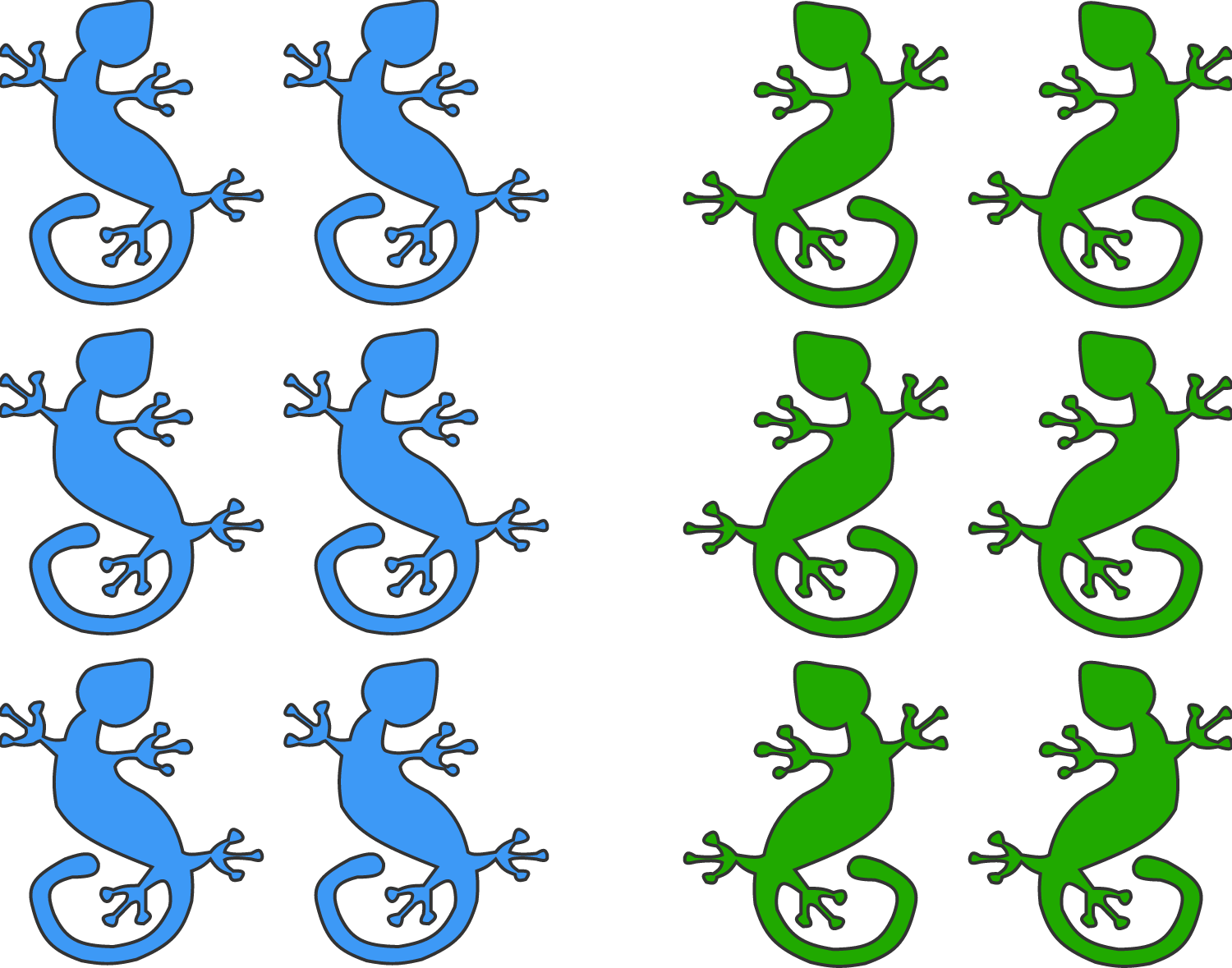Probability

# Discrete Probability: Level 3 Challenges

I have a bag containing 20 red balls and 16 blue balls. I uniformly randomly take balls out from the bag without replacement until all balls of a color have been removed. If the probability that the last ball I took was red can be represented as $\frac{p}{q}$, where $p$ and $q$ are coprime positive integers, find $p+q$.

Diana plays a special version of minesweeper on a square-shaped game field with $n\times n$ smaller squares, where $n$ is an integer greater than or equal to 4. First, she clicks any square, and then the game generates a mine in every non-clicked square, except one. Also, a number (an integer between 0 and 8) appears in the square Diana clicked, telling how many mines there are next to the square (in the small squares horizontally, vertically or diagonally touching it).Diana's goal is to hit the only mineless square without hitting any mines before that. If Diana plays the best way she can, what is the probability she will succeed?

Seven balls of different weights are randomly painted red, orange, yellow, green, blue, indigo and violet, each ball being painted a distinct color.

The green ball is found to be heavier than the blue ball, and the red ball is found to be heavier than the yellow ball.

Given just this information, if the probability that the red ball is heavier than the blue ball is $\dfrac{a}{b}$, where $a,b$ are coprime positive integers, find $a + b$.Three 6-sided fair dice are rolled together. Let $P(n)$ denotes the probability of obtaining a total sum of $n$. What is the relationship between $P(9)$ and $P(10)$?There are 12 chameleons: 6 are blue, 6 are green.

They randomly form four groups of three. In any group, if there is only one chameleon of a particular color, it changes to the color of the other two. Otherwise, chameleons don't change color.

After this random grouping, if the probability that there will be nine blue chameleons and three green ones is $\dfrac{a}{b}$, where $a$ and $b$ are coprime positive integers, what is $a+b$?

×